# 教你如何用 Keras 搭建分类神经网络

• 2021 年 11 月 12 日
• 本文字数：5482 字

阅读完需：约 18 分钟

​​摘要：本文主要通过 Keras 实现了一个分类学习的案例，并详细介绍了 MNIST 手写体识别数据集。

# 一.什么是分类学习

## 1.Classification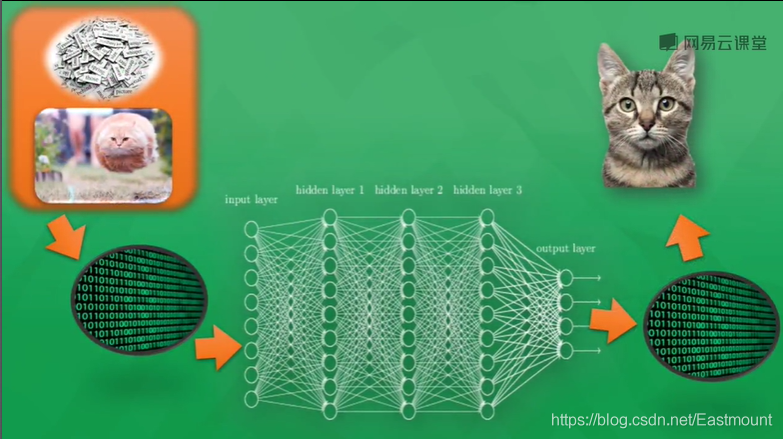• 训练。给定一个数据集，每个样本都包含一组特征和一个类别信息，然后调用分类算法训练模型。

• 预测。利用生成的模型对新的数据集（测试集）进行分类预测，并判断其分类结果。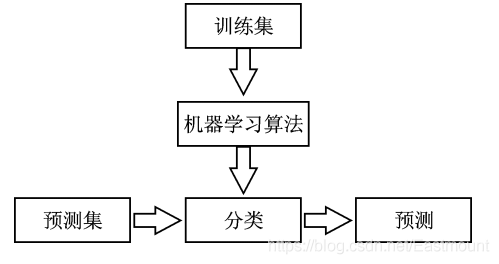## 2.MNIST

MNIST 是手写体识别数据集，它是非常经典的一个神经网络示例。MNIST 图片数据集包含了大量的数字手写体图片，如下图所示，我么可以尝试用它进行分类实验。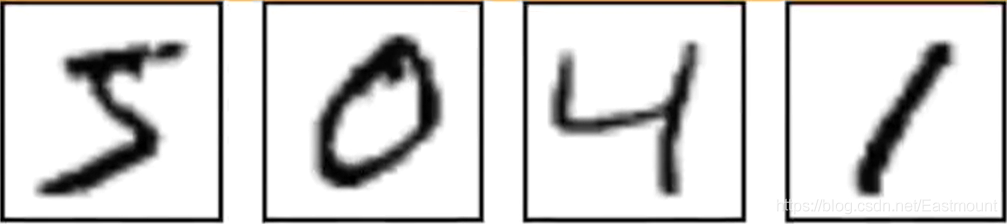MNIST 数据集是含标注信息的，上图分别表示数字 5、0、4 和 1。该数据集共包含三部分：

• 训练数据集：55,000 个样本，mnist.train

• 测试数据集：10,000 个样本，mnist.test

• 验证数据集：5,000 个样本，mnist.validation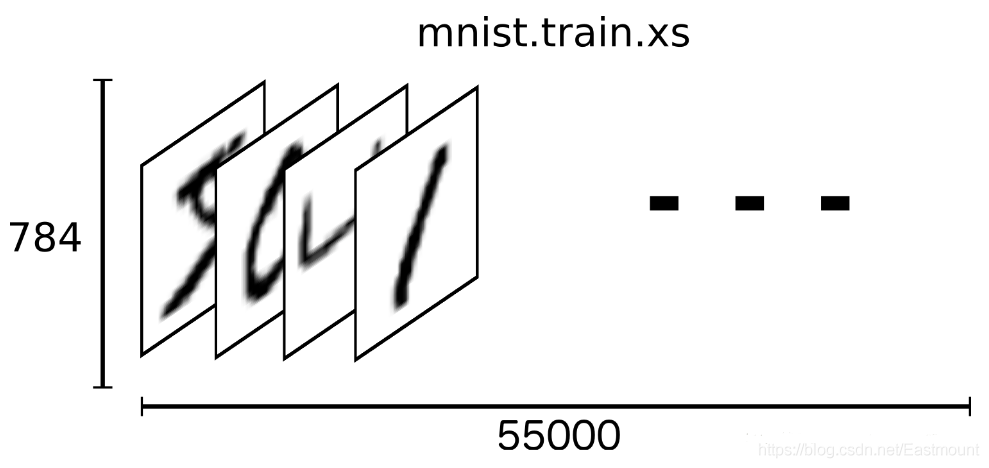MNIST 数据集中的一个样本数据包含两部分内容：手写体图片和对应的 label。这里我们用 xs 和 ys 分别代表图片和对应的 label，训练数据集和测试数据集都有 xs 和 ys，使用 mnist.train.images 和 mnist.train.labels 表示训练数据集中图片数据和对应的 label 数据。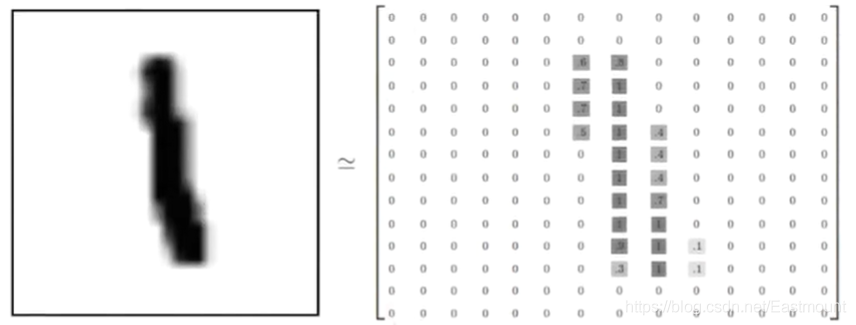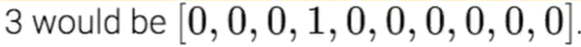mnist.train.labels 是一个 55000*10 的二维数组，如下图所示。它表示 55000 个数据点，第一个数据 y 表示 5，第二个数据 y 表示 0，第三个数据 y 表示 4，第四个数据 y 表示 1。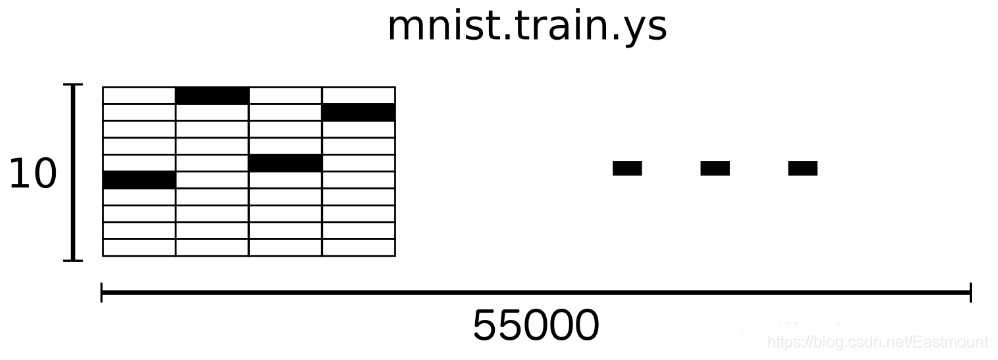# 二.Keras 实现 MNIST 分类

import numpy as npfrom keras.datasets import mnistfrom keras.utils import np_utilsfrom keras.models import Sequentialfrom keras.layers import Dense, Activationfrom keras.optimizers import RMSprop

• X_train.reshape(X_train.shape,-1) / 255

• np_utils.to_categorical(y_train,nb_classes=10)

# 下载MNIST数据 # X shape(60000, 28*28) y shape(10000, )(X_train, y_train), (X_test, y_test) = mnist.load_data()# 数据预处理X_train = X_train.reshape(X_train.shape, -1) / 255  # normalizeX_test = X_test.reshape(X_test.shape, -1) / 255     # normalize# 将类向量转化为类矩阵  数字 5 转换为 0 0 0 0 0 1 0 0 0 0 矩阵y_train = np_utils.to_categorical(y_train, num_classes=10)y_test = np_utils.to_categorical(y_test, num_classes=10)

• model = Sequential()

• from keras.layers importDense, Activation

• from keras.optimizersimport RMSprop

• 第一层为 Dense(32, input_dim=784)，它将传入的 784 转换成 32 个输出

• 该数据加载一个激励函数 Activation(‘relu’)，并转换成非线性化数据

• 第二层为 Dense(10)，它输出为 10 个单位。同时 Keras 定义神经层会默认其输入为上一层的输出，即 32（省略）

• 接着加载一个激励函数 Activation(‘softmax’)，用于分类

# Another way to build your neural netmodel = Sequential([        Dense(32, input_dim=784),  # 输入值784(28*28) => 输出值32        Activation('relu'),        # 激励函数 转换成非线性数据        Dense(10),                 # 输出为10个单位的结果        Activation('softmax')      # 激励函数 调用softmax进行分类        ])# Another way to define your optimizerrmsprop = RMSprop(lr=0.001, rho=0.9, epsilon=1e-08, decay=0.0) #学习率lr# We add metrics to get more results you want to see# 激活神经网络model.compile(        optimizer = rmsprop,                 # 加速神经网络        loss = 'categorical_crossentropy',   # 损失函数        metrics = ['accuracy'],               # 计算误差或准确率        )

print("Training")model.fit(X_train, y_train, nb_epoch=2, batch_size=32)    # 训练次数及每批训练大小print("Testing")loss, accuracy = model.evaluate(X_test, y_test)print("loss:", loss)print("accuracy:", accuracy)

# -*- coding: utf-8 -*-"""Created on Fri Feb 14 16:43:21 2020 @author: Eastmount CSDN YXZO(∩_∩)O Wuhan Fighting!!!"""import numpy as npfrom keras.datasets import mnistfrom keras.utils import np_utilsfrom keras.models import Sequentialfrom keras.layers import Dense, Activationfrom keras.optimizers import RMSprop#---------------------------载入数据及预处理---------------------------# 下载MNIST数据 # X shape(60000, 28*28) y shape(10000, )(X_train, y_train), (X_test, y_test) = mnist.load_data()# 数据预处理X_train = X_train.reshape(X_train.shape, -1) / 255  # normalizeX_test = X_test.reshape(X_test.shape, -1) / 255     # normalize# 将类向量转化为类矩阵  数字 5 转换为 0 0 0 0 0 1 0 0 0 0 矩阵y_train = np_utils.to_categorical(y_train, num_classes=10)y_test = np_utils.to_categorical(y_test, num_classes=10)#---------------------------创建神经网络层---------------------------# Another way to build your neural netmodel = Sequential([        Dense(32, input_dim=784),  # 输入值784(28*28) => 输出值32        Activation('relu'),        # 激励函数 转换成非线性数据        Dense(10),                 # 输出为10个单位的结果        Activation('softmax')      # 激励函数 调用softmax进行分类        ])# Another way to define your optimizerrmsprop = RMSprop(lr=0.001, rho=0.9, epsilon=1e-08, decay=0.0) #学习率lr# We add metrics to get more results you want to see# 激活神经网络model.compile(        optimizer = rmsprop,                 # 加速神经网络        loss = 'categorical_crossentropy',   # 损失函数        metrics = ['accuracy'],               # 计算误差或准确率        )#------------------------------训练及预测------------------------------print("Training")model.fit(X_train, y_train, nb_epoch=2, batch_size=32)    # 训练次数及每批训练大小print("Testing")loss, accuracy = model.evaluate(X_test, y_test)print("loss:", loss)print("accuracy:", accuracy)

​运行代码，首先会下载 MNIT 数据集。

Using TensorFlow backend.Downloading data from https://s3.amazonaws.com/img-datasets/mnist.npz11493376/11490434 [==============================] - 18s 2us/step

​接着输出两次训练的结果，可以看到误差不断减小、正确率不断增大。最终测试输出的误差 loss 为“0.185575”，正确率为“0.94690”。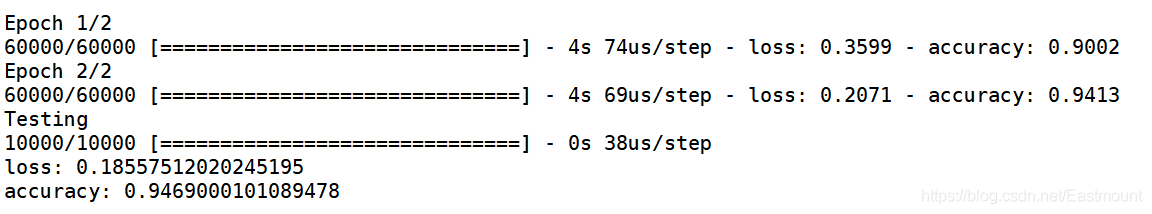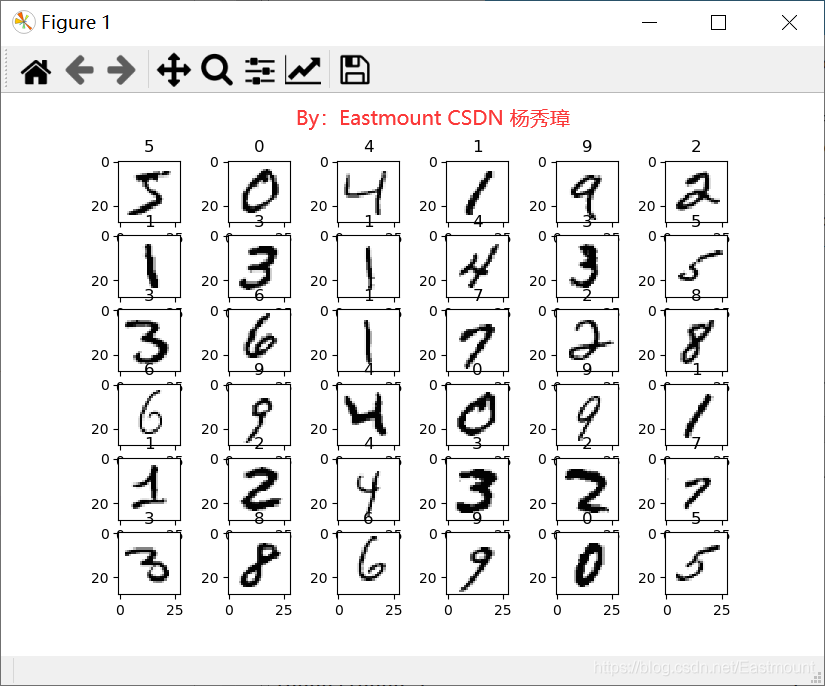# -*- coding: utf-8 -*-"""Created on Fri Feb 14 16:43:21 2020 @author: Eastmount CSDN YXZO(∩_∩)O Wuhan Fighting!!!"""import numpy as npfrom keras.datasets import mnistfrom keras.utils import np_utilsfrom keras.models import Sequentialfrom keras.layers import Dense, Activationfrom keras.optimizers import RMSpropimport matplotlib.pyplot as pltfrom PIL import Image#---------------------------载入数据及预处理---------------------------# 下载MNIST数据 # X shape(60000, 28*28) y shape(10000, )(X_train, y_train), (X_test, y_test) = mnist.load_data()#------------------------------显示图片------------------------------def show_mnist(train_image, train_labels):    n = 6    m = 6    fig = plt.figure()    for i in range(n):        for j in range(m):            plt.subplot(n,m,i*n+j+1)            index = i * n + j #当前图片的标号            img_array = train_image[index]            img = Image.fromarray(img_array)            plt.title(train_labels[index])            plt.imshow(img, cmap='Greys')    plt.show()show_mnist(X_train, y_train)# 数据预处理X_train = X_train.reshape(X_train.shape, -1) / 255  # normalizeX_test = X_test.reshape(X_test.shape, -1) / 255     # normalize# 将类向量转化为类矩阵  数字 5 转换为 0 0 0 0 0 1 0 0 0 0 矩阵y_train = np_utils.to_categorical(y_train, num_classes=10)y_test = np_utils.to_categorical(y_test, num_classes=10)#---------------------------创建神经网络层---------------------------# Another way to build your neural netmodel = Sequential([        Dense(32, input_dim=784),  # 输入值784(28*28) => 输出值32        Activation('relu'),        # 激励函数 转换成非线性数据        Dense(10),                 # 输出为10个单位的结果        Activation('softmax')      # 激励函数 调用softmax进行分类        ])# Another way to define your optimizerrmsprop = RMSprop(lr=0.001, rho=0.9, epsilon=1e-08, decay=0.0) #学习率lr# We add metrics to get more results you want to see# 激活神经网络model.compile(        optimizer = rmsprop,                 # 加速神经网络        loss = 'categorical_crossentropy',   # 损失函数        metrics = ['accuracy'],               # 计算误差或准确率        )#------------------------------训练及预测------------------------------print("Training")model.fit(X_train, y_train, nb_epoch=2, batch_size=32)    # 训练次数及每批训练大小print("Testing")loss, accuracy = model.evaluate(X_test, y_test)print("loss:", loss)print("accuracy:", accuracy)## 评论